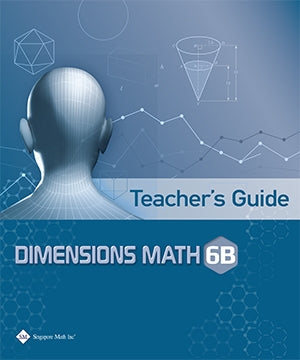# Singapore Math Dimensions Math Teacher's Guide 6B

Dimensions Math® Textbook 6B

Chapter 8: Algebraic Expressions

8.1 Writing and Evaluating Algebraic Expressions

A. Use of Letters
B. Evaluating Algebraic Expressions
C. Word Problems

8.2 Simplifying Algebraic Expressions

In A Nutshell

Chapter 9: Equations and Inequalities

9.1 Equations

A. Algebraic Equations
B. Balancing Equations

9.2 Inequalities

A. Algebraic Inequalities
B. Graphing Inequalities Using a Number Line

In A Nutshell
Problem Solving Corner

Chapter 10: Coordinates and Graphs

10.1 The Coordinate Plane
10.2 Distance between Coordinate Pairs
10.3 Changes in Quantities

A. Independent and Dependent Variables
B. Representing Relationships between Variables
C. Observing Relations between Variables with Graphs

In A Nutshell

Chapter 11: Area of Plane Figures

11.1 Area of Rectangles and Parallelograms
11.2 Area of Triangles

A. Finding Area of a Triangle
B. Areas Involving Parallelograms and Triangles

11.3 Area of Trapezoids

In A Nutshell

Chapter 12: Volume and Surface Area of Solids

12.1 Volume of Rectangular Prisms

A. Cubes and Cuboids
B. Volume of Liquids

12.2 Surface Area of Prisms

A. Surface Area of Rectangular Prisms
B. Surface Area of Triangular Prisms
C. Metric Conversions and Volume

In A Nutshell
Problem Solving Corner

Chapter 13: Displaying and Comparing Data

13.1 Statistical Variability

A. Statistical Questions
B. Measures of Center

13.2 Displaying Numerical Data

A. Dot Plots
B. Histograms

13.3 Measures of Variability and Box Plots

A. Range
B. Mean Absolute Deviation
C. Interquartile Range
D. Box Plot

In A Nutshell

Dimensions Math® G6-8 Textbooks are designed for middle school students. Developed in collaboration between Star Publishing and Singapore Math Inc®, this series follows the Singapore Mathematics Framework and covers the topics in the Common Core State Standards.

The emphasis of this series is on empowering the students to learn mathematics effectively and independently. Depending on the topics covered, different approaches are adopted for the presentation of concepts to facilitate easy understanding by students to internalize concepts and instill in them an interest to explore the topics further.

Each book includes appropriate examples, class activities and diagrams to understand the concepts and apply them. IT skills and values are incorporated as appropriate.

Some features of this series include the following:

1. Class activities which allow the student to learn mathematics through discovery. Some of the class activities make use of Geometer’s Sketchpad (available at keypress.com).
2. Worked examples are followed by a similar question (Try It!) so that students can check if they have understood the concepts presented earlier.
3. An exercise for each lesson including questions in the following sequence:
• Basic Practice (simple questions involving a direct application of the concepts)
• Further Practice (more challenging questions on direct application)
• Maths@Work (questions that apply mathematical concepts to real-life situations)
• Brainworks (questions involving higher order thinking or an open-ended approach to problems)
4. Each chapter is followed by a review exercise; the Extend Your Learning Curve activity encourages students to explore mathematical concepts further or apply mathematics in real-life situations, with some questions requiring sentence or paragraph answers to encourage students to reflect on their learning experiences.
5. The right margins contain remarks on important information, concepts or definitions covered earlier related to the current material to help students recall them, discussion questions, interesting puzzles or facts that are related to mathematics, and some website references.

The answer key at the back of the book provides answers to the Try It! and the problems in the exercises for the Basic PracticeFurther Practice, and Maths@Work questions. It does not include answers to the class activities or the Extend Your Learning Curve activities.